﻿ The Static Analysis of the Truss

### The Static Analysis of the Truss

Pavol Lengvarský, Jozef Bocko

American Journal of Mechanical Engineering

## The Static Analysis of the Truss

Pavol Lengvarský1,, Jozef Bocko1

1Department of Applied Mechanics and Mechanical Engineering, Faculty of Mechanical Engineering, Technical University of Košice, Košice, Slovakia

### Abstract

In this paper the static analysis of the truss is investigated. The analytical and computational method of the roof structures are presented. At the beginning, the analytical method is used for determination of values of external supports, axial forces and principal stresses in truss. Then the computational method is used for the solution of the same problems. The roof structure is modeled by the finite element method, where the bar members are represented by the truss elements. The finite element model loaded by external forces, without and with self-weight of structure is computed. At the end, the analytical and computational buckling analysis for the trusses with maximal compressive axial force is performed. The comparison of results of analytical and computational method shows that all methods are accurate enough, but of course computational method is faster and more adaptive for any subsequent changes.

• Pavol Lengvarský, Jozef Bocko. The Static Analysis of the Truss. American Journal of Mechanical Engineering. Vol. 4, No. 7, 2016, pp 440-444. http://pubs.sciepub.com/ajme/4/7/38
• Lengvarský, Pavol, and Jozef Bocko. "The Static Analysis of the Truss." American Journal of Mechanical Engineering 4.7 (2016): 440-444.
• Lengvarský, P. , & Bocko, J. (2016). The Static Analysis of the Truss. American Journal of Mechanical Engineering, 4(7), 440-444.
• Lengvarský, Pavol, and Jozef Bocko. "The Static Analysis of the Truss." American Journal of Mechanical Engineering 4, no. 7 (2016): 440-444.

 Import into BibTeX Import into EndNote Import into RefMan Import into RefWorks

12345
Prev Next

### 1. Introduction

A truss consists of straight members (links) connected at joints (pins) [1, 2, 3]. Cross-section area of members can be different, but it is constant along a member. In case, the joints are represented by pin connections without friction, the members are loaded by pure axial loading. Such assumption is applicable if the length of member is significantly bigger than its cross-section area. In such case, the real connection of members (bolt connection, welded connection, rivet connection, and so on) can be considered to be ideal joint. Typical example of a truss is a bridge structure given in Figure 1 or roof structure given in Figure 2 [1, 2, 3].Download asVeiw figureFigures index
Figure 1. The truss bridge structureDownload asVeiw figureFigures index
Figure 2. The truss roof structure

The forces are assumed to be applied to the joints. Accordingly, distributed loading acting to truss (weights of members of the truss, effect of wind and so on) has to be transformed into joints, too [1, 2, 3].

The members of truss represent internal constraints between two mass point – joints. These constraints remove the joint one degree of freedom in the axis of a bar. If we remove internal constraints between mass points, we have to replace them by internal reactions in bars. They are assigned by letters Ni, where i is a number of bars in the truss [1, 2, 3].

An individual member can be loaded by axial force in tension or compression. Their magnitude and orientation depends on geometry of truss, external loading and support of whole truss [1, 2, 3].

Positive axial force (tension) is oriented outside of cutted bar (or joint). Negative axial force (compression) is oriented inside of cutted bar (or joint). In computations we will presupposed to have positive forces in all members.

By removing truss members and by their replacement with axial forces as well as by removing external constraints and their replacement by reactions we get a system of mass points in a plane. Such system is in static equilibrium if it is in equilibrium every point of system, i.e. there are fulfilled static equilibrium conditions [1, 2, 3].

Some applications of trusses and their assemblies can be seen in [4, 5, 6, 7].

### 2. Static Analysis of Trusses

2.1. Methods of Static Analysis of Trusses

The methods of statics allow to solve only statically determinate trusses. These methods allow to solve external reactions and internal forces in members. If we want to compute deformations or statically indeterminate structures, we have to use relations from the theory of elasticity. In the next, we will solve statically determinate trusses by means of statics. We will determine static determinacy, external reactions and internal forces in members [1, 2, 3].

The methods of solutions of trusses are divided into three basic groups [1, 2, 3]:

- analytical,

- graphical,

- computational.

Every group of method consist of several steps of solution. Application of computers significantly enhanced computational procedures. Computer based methods, e.g. the finite element method (FEM) pushed back using of graphical methods (e.g. Cremona method, Wiliott method and so on). In order to explain physical principles of problem solution we explain in the next two most important analytic methods: the method of joints and the method of sections (Ritter) [1, 2, 3].

2.1.1. Method of Joints

Principle of method is based on conditions of equilibrium of the systems of mass points. In this case, the mass point is represented by released joint in which act known external and unknown internal forces (in members) [1, 2, 3].

Procedure of computation in case of plane truss [1, 2, 3].

We release truss system from external supports and from the equilibrium conditions for whole truss we compute external reactions and from equilibrium conditions for released joints we compute unknown axial forces in members. We start with the joint in which we know external forces (or reactions) and where are maximum two unknown axial forces.

The members in which are zero forces we called zero members. Even if those forces are not loaded, they are necessary for ensuring the stability of truss.

2.1.2. Method of Sections (Ritter)

The principle of method comes out from equilibrium conditions of a part of truss which is separated by thought cut by maximal three members whose acting line do not cross one point. The axial forces in sectioned members and external forces that act on separated part of structure have to create an equilibrium system. Moment-static equilibrium conditions of separated part of structure has to be written with respect to points that are cross sections of two sectioned members. In some structures such a possibility does not exist and in such case we have to use static force equilibrium conditions .

2.2. Roof Model

The symmetric roof construction (Figure 3) with pitch α=14.036° is consisted from two types of trusses with steel angle section (Figure 4). The trusses are made of steel S235 with yield strength 235 MPa and Young’s modulus 200 000 MPa. The circuit trusses consist of angles L 100x100x10 with section area equal A = 1920mm2 and internal trusses are angles L 50x50x6 with section area equal A = 569 mm2. The basic dimensions of the angle section (Figure 4) are given in Table 1.Download asVeiw figureFigures index
Figure 3. The model of the roof structureDownload asVeiw figureFigures index
Figure 4. Angle section of bar

#### Table 1. The parameters of steel angles

The construction is loaded by force in joints equal F = 2 kN, which is the maximal load computed according to STN EN 1991-1-3.

2.2.1. Analytical Solution of Trusses

In the analytical solution we use the method of joints for computation of the axial forces in bars. At the beginning, we determine the static determinancy (external and internal) of truss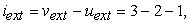(1)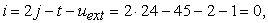(2)

where vext is number of degrees of freedom of the whole construction, uext is number of degrees of freedom taken away by external support, j is number of joints and t is number of bar members.

Finally, we determine the value of external supports (Figure 5) from equilibrium equations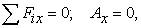(3)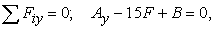(4)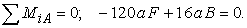(5)

From these equations we get reaction forces Ax = 0 kN and Ay = B = 15 kN, the resulting reaction A is equal to Ay.Download asVeiw figureFigures index
Figure 5. The release of the truss system

Now we compute unknown internal forces in individual members of roof construction. We show this procedure of computation only for one joint (Figure 6) because of a large number of equations. We write equilibrium conditions for joint A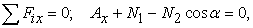(6)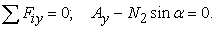(7)

From these conditions we get axial forces equal N1 = 60 kN and N2 = 61,85 kN.Download asVeiw figureFigures index
Figure 6. Forces in joint A

The stresses in the bars we can be compute by following equation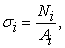(8)

where Ni is the axial force in the bar and Ai is its section area.

The all computed axial forces and stresses of the trusses are given in Table 2.

#### Table 2. The computed values of the axial forces and the principal stresses in trusses

2.2.2. Computational Solution of Trusses

The roof construction is computed using finite element method. The model is created from bar elements and from the boundary conditions and forces applied on the model (Figure 7). The stresses, deformations, axial forces in truss members and reaction forces in supports are obtained from computation. In this solution we do not consider the self-weight of the roof construction.Download asVeiw figureFigures index
Figure 7. The model of roof structure with load and boundary conditions

The reaction forces Ax = 0 kN and Ay = B = 15 kN are computed by the finite element method and they are the same as value of reactions computed by analytical method.

The computed principal stresses are shown in Figure 8. The maximal principal stress is 32.21 MPa in the bar number 2.Download asVeiw figureFigures index
Figure 8. The principal stresses of the roof construction in MPa

The maximal nodal displacement (Figure 9) in vertical direction is equal 8.65 mm.Download asVeiw figureFigures index
Figure 9. The maximal displacement of the roof construction in meters

Now, we perform the same computation considering the self-weight of the roof construction. The reaction forces in supports are Ax = 0 kN and Ay = B =18.03 kN. The computed principal stresses of the loaded roof construction and with the self-weight are shown in Figure 10.Download asVeiw figureFigures index
Figure 10. The principal stresses of the roof construction in MPa

The maximal deformation (Figure 11) in vertical direction for loaded model and with its self-weight is equal 10.33 mm.Download asVeiw figureFigures index
Figure 11. The maximal desplacement of the roof construction in meters

The comparison of computed axial forces and stresses of the trusses are given in Table 3.

#### Table 3. The obtained values of the axial forces and the principal stresses in trusses

The magnitudes of axial forces and stresses from analytical and computational methods are in good agreement.

### 3. Buckling Analysis of Trusses

Because the maximal compressive stresses are in bars with numbers 2 and 22, the buckling analysis for bar 2 and 22 is performed by the analytical (Euler’s column) as well as computational (FEM) method. The trusses can buckle when the critical buckling force is reached. The maximal axial force in truss 2 is F = 73.42 kN and in truss 22 is F = 9.55 kN. The simply-simply support (Figure 12) is considered for the buckling analysis.Download asVeiw figureFigures index
Figure 12. Boundary conditions applied to the member
3.1. Euler’s Column Buckling

The critical buckling force of bar is computed by Euler’s column formula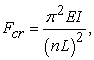(9)

where Fcr, E, I, n and L is the critical buckling force, the Young’s modulus, the moment of inertia, the coefficient that depends on the boundary conditions and the length of bar, respectively .

For the bar 2 is the critical buckling force computed as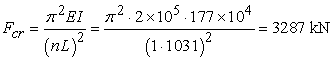(10)

and for the bar 22 is the critical buckling force computed as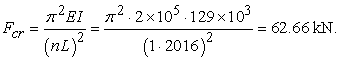(11)
3.2. Computational Buckling

The computational buckling was accomplished by the finite element method. The boundary conditions (simply-simply supported) were assigned and the buckling analysis was performed by the finite element method. The computed critical buckling load is Fcr = 3251.36 kN for truss 2 and Fcr = 62.98 kN for truss 22. The shape of buckling mode of truss number 2 is shown on Figure 13.

Both, analytically and computational computed values of critical buckling force are higher as the axial compressive forces in these bars, so the bars cannot buckle.Download asVeiw figureFigures index
Figure 13. The buckling mode of the bar

### 4. Conclusion

The static analysis of the trusses was investigated. The short introduction to the analytical method was presented. The practical example of roof construction was computed by analytical as well as computational method. At the beginning, the roof construction was replaced by truss elements. In the analytical solution the method of joints was used for determination the external support forces, axial forces in the bars and the principal stresses in trusses. In the computational solution the finite element method was used for the same investigation. The truss members in FEM were represented by truss elements. The finite element model of the roof construction without and with self-weight was computed. The analytical and computational linear buckling analysis for the trusses with maximal compressive axial force was performed, too. The computed stresses were smaller than yield stress of material, so it is possible use steel trusses with smaller angle section. The computed critical buckling forces for bars were higher than the computed compressive axial force in trusses. The comparison of the results shows that the methods for analytical and computational solution of the trusses are accurate enough.

### Acknowledgements

This research was supported by a grant from the Slovak Grant Agency VEGA No. 1/0731/16 - Development of Modern Numerical and Experimental Methods of Mechanical System Analysis.

### References

  Meriam, J.L. and Kraige, L.G., Engineering Mechanics: Statics, Wiley, Texas, 2011.In article  Cherchuk, R.N., Method of Joints (Easy Way), Amazon Digital Services LLC, 2014, [E-book] Available: Amozon.com.In article  Bocko, J. and Delyová, I., Statics, Technical University of Košice, Košice, 2015.In article PubMed  PubMed  ÉLESZTÖS, P. and ÉCSI, L., “Parametrical study of free vibration and plastic collapse of clamped beam,” Acta Mechanica Slovaca, 14, 58-63, 2010.In article  Togan, V., Durmaz, M. and Daloglu, A., “Optimization of roof trusses under snow loads given in Turkish Codes,” Engineering Structures, 28, 1019-1027, 2006.In article  Ronald, W., “Structural performance of light-frame truss-roof assemblies,” in Proceedings of the international engineering conference, Louisiana State University, 263-268.In article PubMed  Frans, R. and Arfiadi, Y., “Sizing, shape and topology optimizations of roof trusses using hybrid genetic algorithms,” in The 2nd International Conference on Sustainable Civil Engineering Structures and Construction Materials, Elsevier, 185-195.In article View Article  Jančo, R., “Numerical and exact solution of buckling load for beam on elastic foundation,” in Sborník vědeckých prací Vysoké školy báňské-Technické univerzity Ostrava, Technical University West Bohemia, 21-26.In article